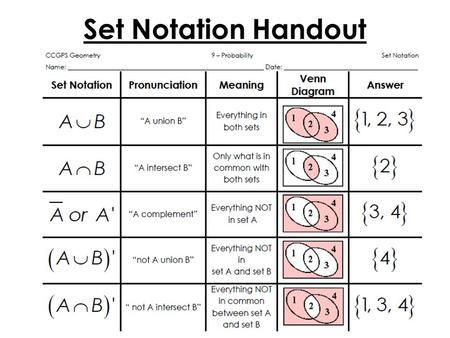# Math background

## Sets

Set a collection of distinct elements:

• $${a, b, c, d}$$ - finite
• $$\mathbb{R}$$ : real numbers - infinite
• $${x \in \mathbb{R}: cos(x) > 1/2}$$ - set with restriction

Notations:

• $$\Omega$$ - universal set(set that contain all objects)
• $$\in$$ - is member of
• $$2 \in \{1, 2, 3, 4\}$$
• $$\notin$$ - is not member of
• $$2 \notin \{1, 3, 5\}$$
• $$A’$$, $$A^c$$, $$\overline{\rm A}$$ - $$A$$ compliment, everything not in $$A$$
• $$x \in S^c if x \in \Omega, x \notin S$$
• $$\emptyset$$ and $$\varnothing$$ or $$\{ \}$$ - empty set
• $$\Omega^c = \emptyset$$
• $$\cup$$ - union, everything in both sets
• $$\{1, 2, 3\} \cup \{3, 4, 5\} = \{1, 2, 3, 4, 5\}$$
• $$x \in S \cup T \leftrightarrow x \in S \, \textsf{or} \> x \in T$$
• $$\cup_{n} S_{n}$$ - union of all $$n$$ sets
• $$\cap$$ - intersection, only what is in common in both sets
• $$\{1, 2, 3\} \cap \{2, 3, 4\} = \{2, 3\}$$
• $$x \in S \cap T \leftrightarrow x \in S \, \textsf{and} \, x \in T$$
• $$\cap_{n} S_{n}$$ - intersection of all $$n$$ sets
• $$\subset$$ - subset of set
• $$\{1, 2, 3\} \subset \{1, 2, 3, 4, 8\}$$
• $$\supset$$ - superset of set
• $$\{1, 2, 3, 4, 8\} \supset \{1, 2, 3\}$$### Sets properties

• $$S \cup T = T \cup S$$
• $$S \cap (T \cup U) = (S \cap T) \cup (S \cap U)$$
• $$(S^c)^c = S$$
• $$S \cup \Omega = \Omega$$
• $$S \cap S^c = \emptyset$$
• $$S \cap \Omega = S$$
• $$S \cup (T \cup U) = (S \cup T) \cup U$$
• $$S \cup (T \cap U) = (S \cup T) \cap (S \cup U)$$

### De Morgan’s laws

• $$(S \cap T)^c = S^c \cup T^c$$
• $$(\cap_{n} S_{n}^c) = \cup_{n} S_{n}^c$$
• $$(S \cup T)^c = S^c \cap T^c$$
• $$(\cup_n S_{n}^c) = \cap_n S_{n}^c$$

### Countable versus uncountable infinite sets

Countable: can be put in 1-1 correspondence with positive integers:

• Positive integers
• Integers: $$0, 1, -1, 2, -2, …$$
• Pairs of positive integers
• Rational numbers $$q$$, with $$0 < q < 1$$, $$1/2, 1/3, 2/3, 1/4, 2/4, …$$

Uncountable: not countable:

• The interval [0, 1]
• The reals
• The plane

## Sequences and their limits

Sequence is some collection of an elements:

• $$a_1, a_2, a_3, …$$
• sequence $$a_1$$
• $$\{a_i\}$$
• formal - function $$f: \mathbb{N} -> S, f(i) = a_i$$

When does a sequence converge:

• If $$a_i \leq a_{i + 1}$$ for all $$i$$, then either:
• the sequence “converges to $$\infty$$
• the sequence converges to some real number $$a$$
• if $$|a_i - a| \leq b_i$$ for all $$i$$, and $$b_i -> 0$$, then $$a_i -> a$$

## Series

### Infinite series

$$\sum_{i=1}^{\infty} a_i = \lim_{n -> \infty} \sum_{i=1}^n a_i$$ (provided limit exists)

• if $$a_i \geq 0$$: limit exists
• if terms $$a_i$$ do not all have the same sign:
• limit need not exist
• limit may exist but be different if we sum in different order
• Fact: limit exists and independent of order of summation if $$\sum_{i=1}^{\infty} |a_i| < \infty$$

### Order of summation in series

only if $$\sum |a_{ij}| < \infty$$, then:

• $$\sum_{i \geq 1, j \geq 1} a_{ij} = \sum_{i=1}^{\infty}(\sum_{j = 1}^{\infty} a_{ij}) = \sum_{j=1}^{\infty}(\sum_{i = 1}^{\infty} a_{ij})$$
• $$\sum_{(i, j) : j \leq i} a_{ij} = \sum_{i=1}^{\infty} \sum_{j=1}^{i} a_{ij} = \sum_{j=1}^{\infty} \sum_{i=j}^{\infty} a_{ij}$$

### Arithmetic progression

\begin{equation*} a_{n}=a_{1}+(n-1)d \end{equation*}
\begin{equation*} a_{n}=a_{m}+(n-m)d \end{equation*}
\begin{equation*} S_n = \frac{n}{2}[2a_1 + (n - 1)d] \end{equation*}
\begin{equation*} S_n = \frac{n}{2}[a_1 + a_n] \end{equation*}
\begin{equation*} \overline{\rm n} = S_n / n \end{equation*}
\begin{equation*} \overline{\rm n} = \frac{a_1 + a_n}{2} \end{equation*}

where:

• $$a_1$$ is the first term of an arithmetic progression.
• $$a_n$$ is the nth term of an arithmetic progression.
• $$d$$ is the difference between terms of the arithmetic progression.
• $$n$$ is the number of terms in the arithmetic progression.
• $$S_n$$ is the sum of n terms in the arithmetic progression.
• $$\overline{\rm n}$$ is the mean value of arithmetic series.

### Geometric progression

\begin{equation*} a_n = ar^{n - 1} \end{equation*}
\begin{equation*} a_n = ra_{n - 1} \end{equation*}
\begin{equation*} S_n = \frac{a_1 (1 - r^n)}{1 - r} \end{equation*}
\begin{equation*} S_n = \frac{a_1 - a_n * r}{1 - r} \end{equation*}
\begin{equation*} where r \ne 1 \end{equation*}

where:

• $$a$$ - initial value
• $$r$$ - common ratio

Simplified: $$S = \sum_{i=0}^{\inf} \alpha^i = 1 + \alpha + \alpha^2 + … = \frac{1}{1 - \alpha}$$, if $$|\alpha| < 1$$

pass

## Other

If $$X$$ is a vector, and $$X \in \{-1, 1\}^{n}$$, then $$X^{T}X == n$$, where $$n$$ is a constant, and $$n == len(X)$$.